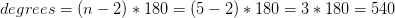## Example Questions

### Example Question #1 : Pentagons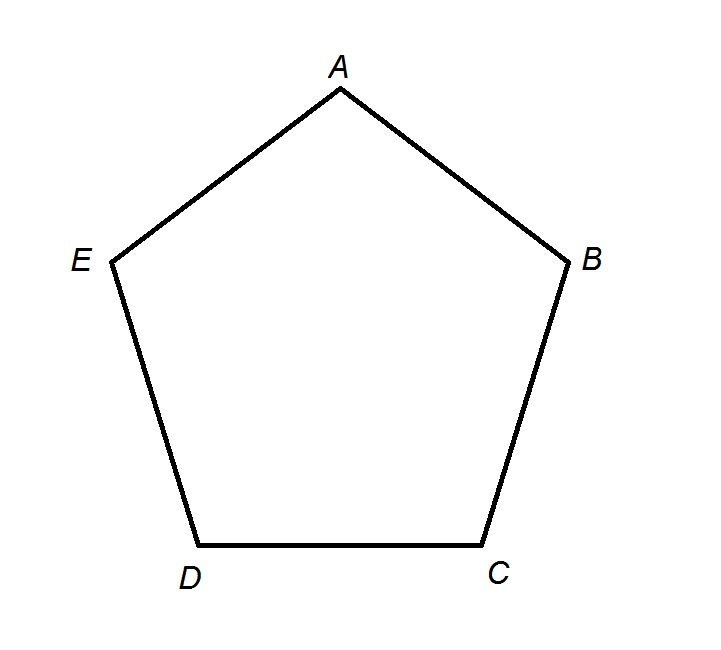The above diagram shows a pentagonal track with perimeter one third of a mile. Adrianne starts at Point A and runs clockwise until she gets halfway between Points D and E. Which of the following choices is closest to the number of feet she runs?

1,100 feet

1,300 feet

1,000 feet

1,400 feet

1,200 feet

1,200 feet

Explanation:

The perimeter of the pentagonal track is one third of a mile; one mile is equal to 5,280 feet, so the perimeter is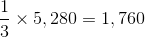feet.

Each side of the pentagon has length one fifth of its perimeter, or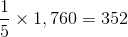feet.

Adrianne runs three and one half sides, or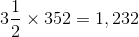feet.

This makes 1,200 feet the closest, and correct, choice.

### Example Question #2 : PentagonsAristotle High School has an unusual track in that it is shaped like a regular pentagon. Each side of the pentagon measures 264 feet.

Benny runs at a steady speed of eight miles an hour for ten minutes, starting at point A and working his way clockwise. When he is finished, which of the following points is he closest to?

Point A

Point B

Point D

Point E

Point C

Point C

Explanation:

Benny runs at a rate of eight miles an hour for ten minutes, or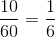hours. The distance he runs is equal to his rate multiplied by his time, so, setting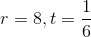in this formula: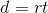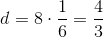miles.

One mile comprises 5,280 feet, so this is equal to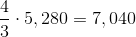feet.

Since each side of the track measures 264 feet, this means that Benny runs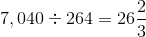sidelengths.

This means Benny runs around the track for 25 sidelengths, which is 5 complete times, back to Point A; he then runs one more complete sidelength to Point B; and, finally, he runsof a sidelength, finishing closest to Point C.

### Example Question #3 : Pentagons

Find the sum of all the angles in a pentagon.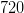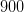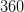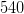Explanation:

To solve, simply use the formula to find the total degrees in a polygon, where n is thenumber of vertices.

In this particular case, a pentagon is a shape that has five sides and thus has five vertices.

Thus,